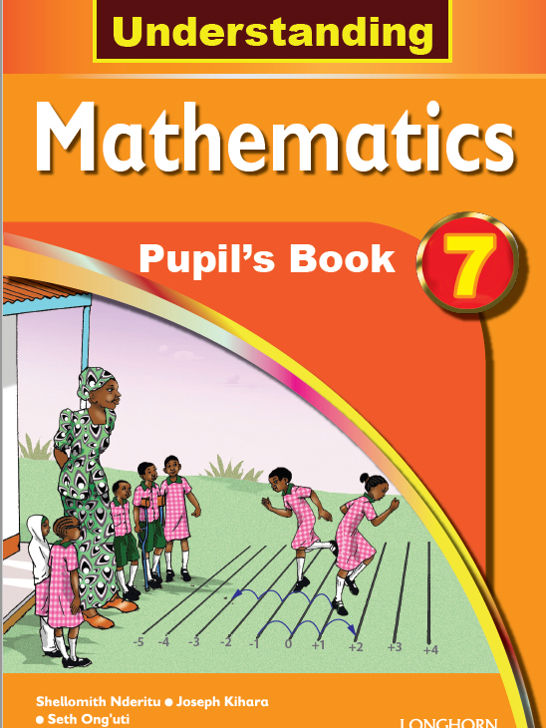Study kit (Mathematics Std 7)

# Mathematics Standard 7##### Authors
Shellomith Nderitu, Joseph Kihara, Seth Ong’uti
##### Exercises kit’s authors
Shellomith Nderitu, Joseph Kihara, Seth Ong’uti
##### Publisher
Longhorn Publishers
##### Included in packages• The study kit contains 32 chapters and teacher’s exercises kit contains 122 exercises.
• ##### Authors
Shellomith Nderitu, Joseph Kihara, Seth Ong’uti
• ##### Exercises kit’s authors
Shellomith Nderitu, Joseph Kihara, Seth Ong’uti
Mathematics
Standard 7
English
• ##### Publisher
Longhorn Publishers

### 1. Numbers. Whole numbers

##### Chapter
1.1. Place value, total value
Free chapter!
1.2. Square of numbers
1.3. Square root and prime factorisation
1.4. Divisibility

### 2. Numbers. Fractions

##### Chapter
2.1. Multiplication of fractions
2.2. Squares of fractions
2.3. Square roots of fraction

### 3. Numbers. Decimals

##### Chapter
3.1. Place value and total value to ten thousandths
3.2. Decimal numbers, place value and total value to millionths
3.3. Rounding off
3.4. Converting decimals to fractions and vice versa
3.5. Square and square roots of decimals

### 4. Number. Percentages

##### Chapter
4.1. Percentage and fractions
4.2. Percentage and decimals

### 5. Operations. Whole numbers

##### Chapter
5.2. Subtraction
5.3. Multiplication
5.4. Division
5.5. Combined operations
5.6. Sequences

### 6. Operations. Fractions

##### Chapter
6.2. Multiplication and division
6.3. Combined operations. Addition and subtraction
6.4. Combined operations. Multiplication and division
6.5. Number sequences